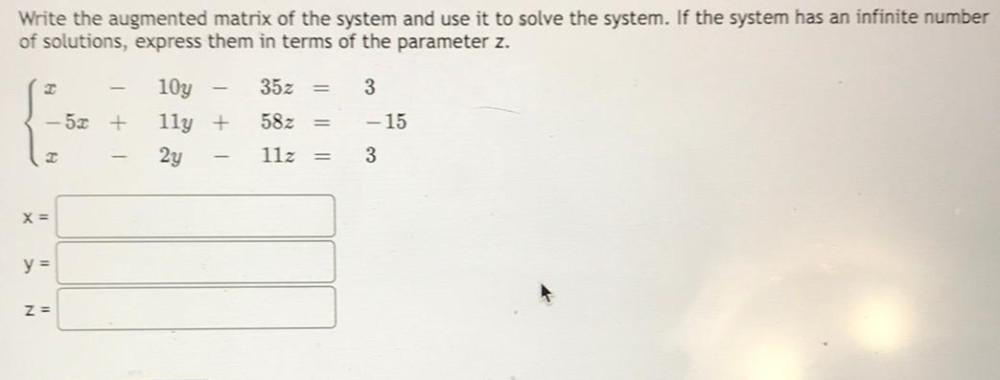Question:

# Write the augmented matrix of the system and use it to solve the system. If the system has an infinite number of solutions, express them in terms of the parameter z. x-10y-35z=3 -5x+11y+58z=-15 x-2y-1Write the augmented matrix of the system and use it to solve the system. If the system has an infinite number of solutions, express them in terms of the parameter z. x-10y-35z=3 -5x+11y+58z=-15 x-2y-11x=3 x=________________ y=________________ z=________________